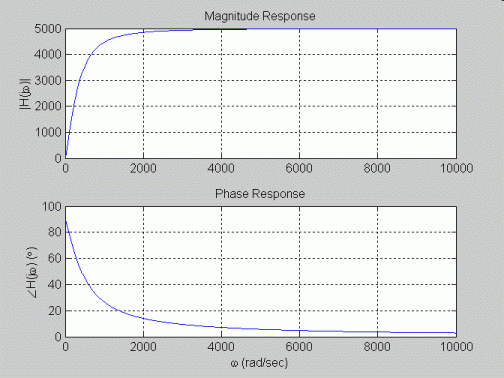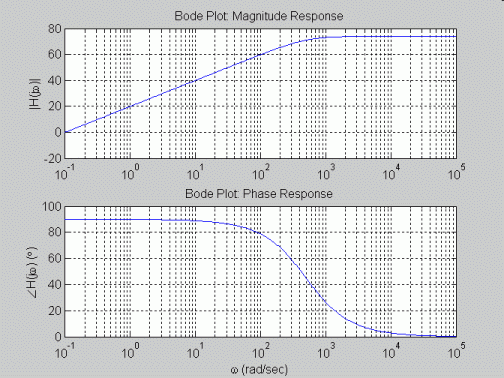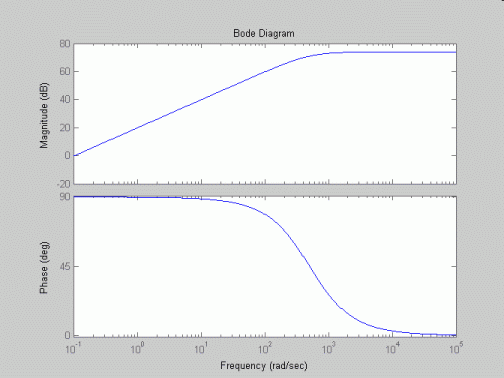EE212 Matlab Example: System Frequency Response

Matlab m-file:

% File Name: example1.m
%
% Description: Matlab m-file for plotting a frequency response of example discussed
% in class under III. B. 12. using
% a) standard plotting and complex number capabilities,
% b) standard plotting and complex number capabilities for generating Bode plots, and
% c) built in Bode plot function.

% Transfer function:           5000jw
%                    H(jw) = ----------
%                             500 + jw

% clear matlab memory and close all open figures
clear all; close all;

%=======================================================================================
% a) plotting of frequency response using standard complex number and plotting functions
%=======================================================================================

% open figure 1 for first frequency response plots
figure(1);

% create vector of 500 equally spaced frequencies from 0.1rad/sec to 10000rad/sec
w = linspace(0.1,10000,500);

% define transfer function
H = 5000*j*w./(500 + j*w);

% divide figure window into two rows, one column, and plot magnitude response in top graph,
% phase response in bottom graph
subplot(2,1,1);
plot(w,abs(H));
grid; ylabel('|H(j\omega)|'); title('Magnitude Response');
subplot(2,1,2);
plot(w,unwrap(angle(H))*180/pi);
grid; xlabel('\omega (rad/sec)'); ylabel('\angleH(j\omega) (\circ)'); title('Phase Response');

%=======================================================================================
% b) plotting of frequency response as a Bode plot using standard complex number
% and plotting functions
%=======================================================================================

% open figure 2 for second frequency response plots
figure(2);

% create vector of 500 logarithmically spaced (i.e., same number of points per decade)
w = logspace(-1,5,500);

% define transfer function
H = 5000*j*w./(500 + j*w);

% divide figure window into two rows, one column, and plot magnitude response in top graph,
% phase response in bottom graph
subplot(2,1,1);
semilogx(w,20*log10(abs(H)));
grid; ylabel('|H(j\omega)|'); title('Bode Plot: Magnitude Response');
subplot(2,1,2);
semilogx(w,unwrap(angle(H))*180/pi);
grid; xlabel('\omega (rad/sec)'); ylabel('\angleH(j\omega) (\circ)'); title('Bode Plot: Phase Response');

%=======================================================================================
% c) plotting of frequency response using Bode function
%=======================================================================================

% open figure 3 for third frequency response plots
figure(3);

% create vector of 500 logarithmically spaced (i.e., same number of points per decade)
w = logspace(-1,5,500);

% define transfer function using coefficients of jw in numerator and denominator
% starting with highest power of jw and working down
numH = [5000 0];
denH = [1 500];

% call bode() to plot frequency response as bode diagrams
bode(numH,denH,w);

Procedure for running m-file: change working directory to where m-file is stored and then enter m-file name at command prompt.

>> pwd

ans =

C:\matlabR12\work

>> cd ../../temp/ee212/
>> dir

. .. example1.m

>> example1
>>

Plots generated: